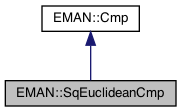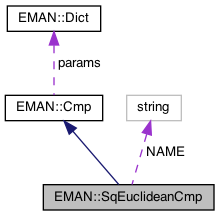EMAN2
EMAN::SqEuclideanCmp Class Reference

Squared Euclidean distance normalized by n between 'this' and 'with'. More...

`#include <cmp.h>`

Inheritance diagram for EMAN::SqEuclideanCmp:[legend]
Collaboration diagram for EMAN::SqEuclideanCmp:[legend]

## Public Member Functions

SqEuclideanCmp ()

float cmp (EMData *image, EMData *with) const
To compare 'image' with another image passed in through its parameters. More...

string get_name () const
Get the Cmp's name. More...

string get_desc () const

TypeDict get_param_types () const
Get Cmp parameter information in a dictionary. More...Public Member Functions inherited from EMAN::Cmp
virtual ~Cmp ()

virtual Dict get_params () const
Get the Cmp parameters in a key/value dictionary. More...

virtual void set_params (const Dict &new_params)
Set the Cmp parameters using a key/value dictionary. More...

static CmpNEW ()

## Static Public Attributes

static const string NAME = "sqeuclidean"Protected Member Functions inherited from EMAN::Cmp
void validate_input_args (const EMData *image, const EMData *with) constProtected Attributes inherited from EMAN::Cmp
Dict params

## Detailed Description

Squared Euclidean distance normalized by n between 'this' and 'with'.

Definition at line 225 of file cmp.h.

## ◆ SqEuclideanCmp()

 EMAN::SqEuclideanCmp::SqEuclideanCmp ( )
inline

Definition at line 228 of file cmp.h.

228{}

Referenced by NEW().

## ◆ cmp()

 float SqEuclideanCmp::cmp ( EMData * image, EMData * with ) const
virtual

To compare 'image' with another image passed in through its parameters.

An optional transformation may be used to transform the 2 images.

Parameters
 image The first image to be compared. with The second image to be comppared.
Returns
The comparison result. Smaller better by default

Implements EMAN::Cmp.

Definition at line 280 of file cmp.cpp.

281{
282 ENTERFUNC;
283 EMData *with = withorig;
284 validate_input_args(image, with);
285
287 int normto = params.set_default("normto",0);
288
289 if (normto) {
290 if (zeromask) with = withorig->process("normalize.toimage",Dict("to",image));
291 else with = withorig->process("normalize.toimage",Dict("to",image,"ignore_zero",0));
292 with->set_attr("deleteme",1);
293 if ((float)(with->get_attr("norm_mult"))<=0) { // This means the normalization inverted the image, a clear probablity of noise bias, so we undo the normalization
294 delete with;
295 with=withorig;
296 }
297 }
298
299 const float *const y_data = with->get_const_data();
300 const float *const x_data = image->get_const_data();
301 double result = 0.;
302 float n = 0.0f;
303 if(image->is_complex() && with->is_complex()) {
304 // Implemented by PAP 01/09/06 - please do not change. If in doubts, write/call me.
305 int nx = with->get_xsize();
306 int ny = with->get_ysize();
307 int nz = with->get_zsize();
308 nx = (nx - 2 + with->is_fftodd()); // nx is the real-space size of the input image
309 int lsd2 = (nx + 2 - nx%2) ; // Extended x-dimension of the complex image
310
311 int ixb = 2*((nx+1)%2);
312 int iyb = ny%2;
313 //
314 if(nz == 1) {
315 // it looks like it could work in 3D, but it does not, really.
316 for ( int iz = 0; iz <= nz-1; iz++) {
317 double part = 0.;
318 for ( int iy = 0; iy <= ny-1; iy++) {
319 for ( int ix = 2; ix <= lsd2 - 1 - ixb; ix++) {
320 size_t ii = ix + (iy + iz * ny)* lsd2;
321 part += (x_data[ii] - y_data[ii])*double(x_data[ii] - y_data[ii]);
322 }
323 }
324 for ( int iy = 1; iy <= ny/2-1 + iyb; iy++) {
325 size_t ii = (iy + iz * ny)* lsd2;
326 part += (x_data[ii] - y_data[ii])*double(x_data[ii] - y_data[ii]);
327 part += (x_data[ii+1] - y_data[ii+1])*double(x_data[ii+1] - y_data[ii+1]);
328 }
329 if(nx%2 == 0) {
330 for ( int iy = 1; iy <= ny/2-1 + iyb; iy++) {
331 size_t ii = lsd2 - 2 + (iy + iz * ny)* lsd2;
332 part += (x_data[ii] - y_data[ii])*double(x_data[ii] - y_data[ii]);
333 part += (x_data[ii+1] - y_data[ii+1])*double(x_data[ii+1] - y_data[ii+1]);
334 }
335
336 }
337 part *= 2;
338 part += (x_data - y_data)*double(x_data - y_data);
339 if(ny%2 == 0) {
340 int ii = (ny/2 + iz * ny)* lsd2;
341 part += (x_data[ii] - y_data[ii])*double(x_data[ii] - y_data[ii]);
342 }
343 if(nx%2 == 0) {
344 int ii = lsd2 - 2 + (0 + iz * ny)* lsd2;
345 part += (x_data[ii] - y_data[ii])*double(x_data[ii] - y_data[ii]);
346 if(ny%2 == 0) {
347 int ii = lsd2 - 2 +(ny/2 + iz * ny)* lsd2;
348 part += (x_data[ii] - y_data[ii])*double(x_data[ii] - y_data[ii]);
349 }
350 }
351 result += part;
352 }
353 n = (float)nx*(float)ny*(float)nz*(float)nx*(float)ny*(float)nz;
354
355 } else { //This 3D code is incorrect, but it is the best I can do now 01/09/06 PAP
356 int ky, kz;
357 int ny2 = ny/2; int nz2 = nz/2;
358 for ( int iz = 0; iz <= nz-1; iz++) {
359 if(iz>nz2) kz=iz-nz; else kz=iz;
360 for ( int iy = 0; iy <= ny-1; iy++) {
361 if(iy>ny2) ky=iy-ny; else ky=iy;
362 for ( int ix = 0; ix <= lsd2-1; ix++) {
363 // Skip Friedel related values
364 if(ix>0 || (kz>=0 && (ky>=0 || kz!=0))) {
365 size_t ii = ix + (iy + iz * ny)* (size_t)lsd2;
366 result += (x_data[ii] - y_data[ii])*double(x_data[ii] - y_data[ii]);
367 }
368 }
369 }
370 }
371 n = ((float)nx*(float)ny*(float)nz*(float)nx*(float)ny*(float)nz)/2.0f;
372 }
373 } else { // real space
374 size_t totsize = (size_t)image->get_xsize()*image->get_ysize()*image->get_zsize();
378 const float *const dm = mask->get_const_data();
379 for (size_t i = 0; i < totsize; i++) {
380 if (dm[i] > 0.5) {
381 double temp = x_data[i]- y_data[i];
382 result += temp*temp;
383 n++;
384 }
385 }
386 }
388 n=0;
389 for (size_t i = 0; i < totsize; i++) {
390 if (x_data[i]==0 || y_data[i]==0) continue;
391 double temp = x_data[i]- y_data[i];
392 result += temp*temp;
393 n++;
394 }
395
396 }
397 else {
398 for (size_t i = 0; i < totsize; i++) {
399 double temp = x_data[i]- y_data[i];
400 result += temp*temp;
401 }
402 n = (float)totsize;
403 }
404 }
405 result/=n;
406
407 EXITFUNC;
408 if (with->has_attr("deleteme")) delete with;
409 float ret = (float)result;
410 if (!Util::goodf(&ret)) return FLT_MAX;
411 return ret;
412}
void validate_input_args(const EMData *image, const EMData *with) const
Definition: cmp.cpp:81
Dict params
Definition: cmp.h:132
Dict is a dictionary to store <string, EMObject> pair.
Definition: emobject.h:385
type set_default(const string &key, type val)
Default setting behavior This can be achieved using a template - d.woolford Jan 2008 (before there wa...
Definition: emobject.h:569
bool has_key(const string &key) const
Ask the Dictionary if it as a particular key.
Definition: emobject.h:511
EMData stores an image's data and defines core image processing routines.
Definition: emdata.h:82
static int goodf(const float *p_f)
Check whether a number is a good float.
Definition: util.h:1112
#define ENTERFUNC
Definition: log.h:48
#define EXITFUNC
Definition: log.h:49
#define dm(i)
Definition: projector.cpp:1606

## ◆ get_desc()

 string EMAN::SqEuclideanCmp::get_desc ( ) const
inlinevirtual

Implements EMAN::Cmp.

Definition at line 237 of file cmp.h.

238 {
239 return "Squared Euclidean distance (sum(a - b)^2)/n.";
240 }

## ◆ get_name()

 string EMAN::SqEuclideanCmp::get_name ( ) const
inlinevirtual

Get the Cmp's name.

Each Cmp is identified by a unique name.

Returns
The Cmp's name.

Implements EMAN::Cmp.

Definition at line 232 of file cmp.h.

233 {
234 return NAME;
235 }
static const string NAME
Definition: cmp.h:256

References NAME.

## ◆ get_param_types()

 TypeDict EMAN::SqEuclideanCmp::get_param_types ( ) const
inlinevirtual

Get Cmp parameter information in a dictionary.

Each parameter has one record in the dictionary. Each record contains its name, data-type, and description.

Returns
A dictionary containing the parameter info.

Implements EMAN::Cmp.

Definition at line 247 of file cmp.h.

248 {
249 TypeDict d;
251 d.put("zeromask", EMObject::INT, "If set, zero pixels in either image will be excluded from the statistics");
252 d.put("normto",EMObject::INT,"If set, 'with' is normalized to 'this' before computing the distance");
253 return d;
254 }

References EMAN::EMObject::EMDATA, EMAN::EMObject::INT, and EMAN::TypeDict::put().

## ◆ NEW()

 static Cmp * EMAN::SqEuclideanCmp::NEW ( )
inlinestatic

Definition at line 242 of file cmp.h.

243 {
244 return new SqEuclideanCmp();
245 }

References SqEuclideanCmp().

## ◆ NAME

 const string SqEuclideanCmp::NAME = "sqeuclidean"
static

Definition at line 256 of file cmp.h.

Referenced by get_name().

The documentation for this class was generated from the following files: